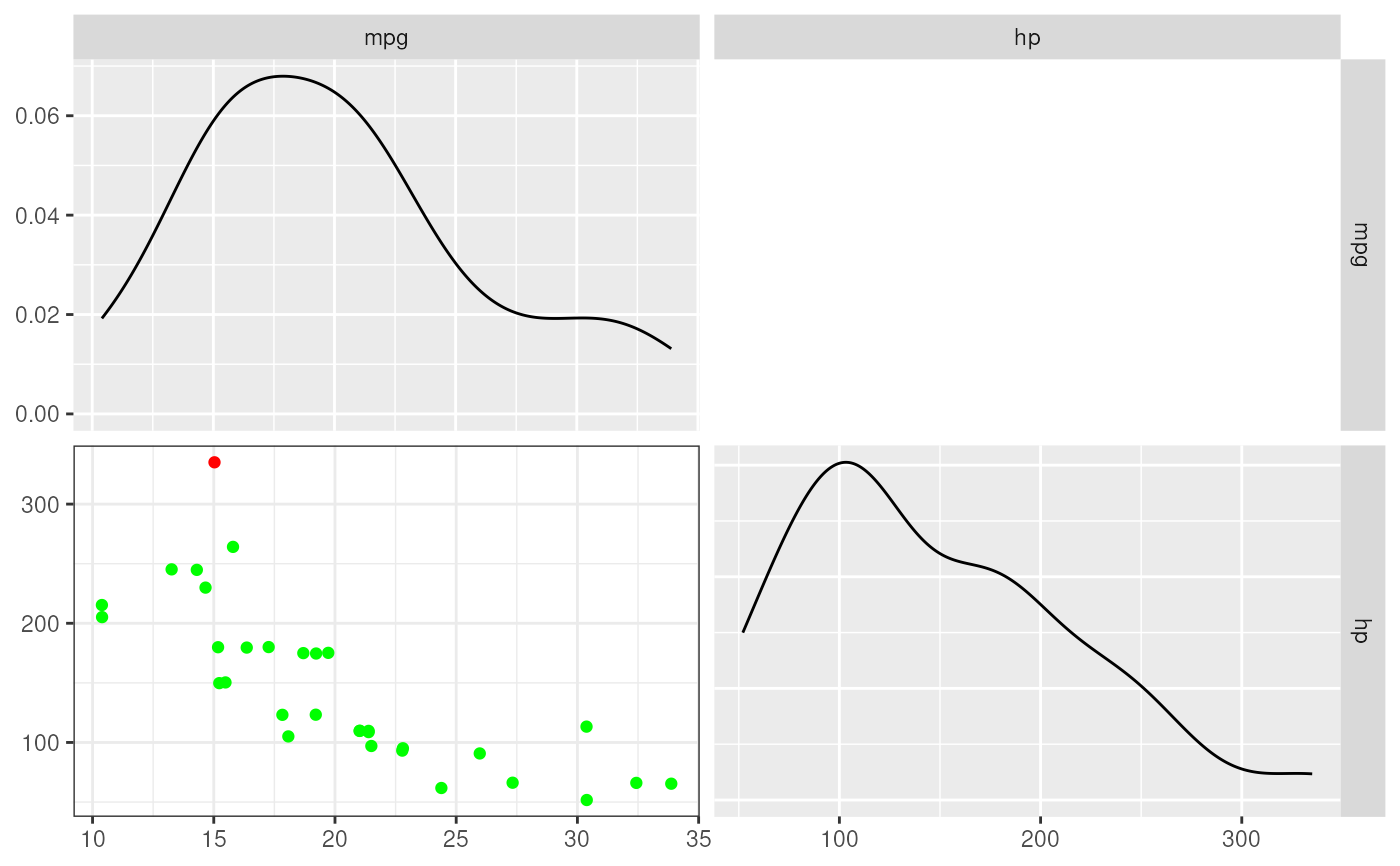This function combines a number of criteria for determining whether a datapoint is an influential case in a regression analysis. It then sum the criteria to compute an index of influentiality. A list of cases with an index of influentiality of 1 or more is then displayed, after which the regression analysis is repeated without those influantial cases. A scattermatrix is also displayed, showing the density curves of each variable, and in the scattermatrix, points that are colored depending on how influential each case is.

regrInfluential(formula, data, createPlot = TRUE)

# S3 method for regrInfluential
print(x, headingLevel = 3, ...)

## Arguments

formula

The formule of the regression analysis.

data

The data to use for the analysis.

createPlot

Whether to create the scattermatrix (requires the GGally package to be installed).

x

Object to print.

The number of hash symbols to prepend to the heading.

...

Additional arguments are passed on to the regr print function.

## Value

A regrInfluential object, which, if printed, shows the influential cases, the regression analyses repeated without those cases, and the scatter matrix.

## Author

Gjalt-Jorn Peters & Marwin Snippe

Maintainer: Gjalt-Jorn Peters gjalt-jorn@userfriendlyscience.com

## Examples


regrInfluential(mpg ~ hp, mtcars);
#>
#> ### Influential cases:
#>
#>               mpg  hp    dfb.1_   dfb.hp    dffit     cov.r   cook.d       hat
#> Maserati Bora  15 335 -1.128627 1.487575 1.580208 0.9791369 1.052231 0.2745929
#>               indexOfInfluentiality
#> Maserati Bora                     5#>
#> #### Regression analyses, repeated without influential cases
#>
#>
#> ##### Omitting all cases marked as influential by 5 criteria
#>
#> Regression analysis for formula: mpg ~ hp
#>
#> Significance test of the entire model (all predictors together):
#>   Multiple R-squared: [0.41, 0.79] (point estimate = 0.6, adjusted = 0.59)
#>   Test for significance: F[1, 30] = 45.46, p < .001
#>
#> Raw regression coefficients (unstandardized beta values, called 'B' in SPSS):
#>
#>             95% conf. int. estimate   se     t     p
#> (Intercept) [26.76; 33.44]    30.10 1.63 18.42 <.001
#> hp          [-0.09; -0.05]    -0.07 0.01 -6.74 <.001
#>
#> Scaled regression coefficients (standardized beta values, called 'Beta' in SPSS):
#>
#>             95% conf. int. estimate   se     t     p
#> (Intercept)  [-0.23; 0.23]     0.00 0.11  0.00     1
#> hp          [-1.01; -0.54]    -0.78 0.12 -6.74 <.001